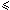Next: Shift Or algorithm Up: ESMAJ Prev: Research with an automaton

# Karp-Rabin algorithm

Main features
• uses an hashing function;
• preprocessing phase in O(m) time complexity and constant space;
• searching phase in O(mn) time complexity;
• O(n+m) expected running time.
Description

Hashing provides a simple method to avoid a quadratic number of character comparisons in most practical situations. Instead of checking at each position of the text if the pattern occurs, it seems to be more efficient to check only if the contents of the window “looks like” the pattern. In order to check the resemblance between these two words an hashing function is used.

To be helpful for the string matching problem an hashing function hash should have the following properties:efficiently computable;highly discriminating for strings;hash(y[j+1 .. j+m]) must be easily computable from hash(y[j .. j+m-1]) and y[j+m]:   hash(y[j+1 .. j+m])= rehash(y[j], y[j+m], hash(y[j .. j+m-1]).

For a word w of length m let hash(w) be defined as follows:
hash(w[0 .. m-1])=(w*2m-1+ w*2m-2+···+ w[m-1]*20) mod q
where q is a large number.

Then, rehash(a,b,h)= ((h-a*2m-1)*2+b) mod q

The preprocessing phase of the Karp-Rabin algorithm consists in computing hash(x). It can be done in constant space and O(m) time.

During searching phase, it is enough to compare hash(x) with hash(y[j .. j+m-1]) for 0j < n-m. If an equality is found, it is still necessary to check the equality x=y[j .. j+m-1] character by character.

The time complexity of the searching phase of the Karp-Rabin algorithm is O(mn) (when searching for am in an for instance). Its expected number of text character comparisons is O(n+m).

The C code

In the following function KR all the multiplications by 2 are implemented by shifts. Furthermore, the computation of the modulus function is avoided by using the implicit modular arithmetic given by the hardware that forgets carries in integer operations. So, q is chosen as the maximum value for an integer.

```#define REHASH(a, b, h) ((((h) - (a)*d) << 1) + (b))

void KR(char *x, int m, char *y, int n) {
int d, hx, hy, i, j;

/* Preprocessing */
/* computes d = 2^(m-1) with
the left-shift operator */
for (d = i = 1; i < m; ++i)
d = (d<<1);

for (hy = hx = i = 0; i < m; ++i) {
hx = ((hx<<1) + x[i]);
hy = ((hy<<1) + y[i]);
}

/* Searching */
j = 0;
while (j <= n-m) {
if (hx == hy && memcmp(x, y + j, m) == 0)
OUTPUT(j);
hy = REHASH(y[j], y[j + m], hy);
++j;
}

}

```
The example

Preprocessing phase

hash[y]=17597

Searching phase

References
• AHO, A.V., 1990, Algorithms for finding patterns in strings. in Handbook of Theoretical Computer Science, Volume A, Algorithms and complexity, J. van Leeuwen ed., Chapter 5, pp 255-300, Elsevier, Amsterdam.
• CORMEN, T.H., LEISERSON, C.E., RIVEST, R.L., 1990. Introduction to Algorithms, Chapter 34, pp 853-885, MIT Press.
• CROCHEMORE, M., HANCART, C., 1999, Pattern Matching in Strings, in Algorithms and Theory of Computation Handbook, M.J. Atallah ed., Chapter 11, pp 11-1--11-28, CRC Press Inc., Boca Raton, FL.
• GONNET, G.H., BAEZA-YATES, R.A., 1991. Handbook of Algorithms and Data Structures in Pascal and C, 2nd Edition, Chapter 7, pp. 251-288, Addison-Wesley Publishing Company.
• HANCART, C., 1993. Analyse exacte et en moyenne d'algorithmes de recherche d'un motif dans un texte, Ph. D. Thesis, University Paris 7, France.
• CROCHEMORE, M., LECROQ, T., 1996, Pattern matching and text compression algorithms, in CRC Computer Science and Engineering Handbook, A. Tucker ed., Chapter 8, pp 162-202, CRC Press Inc., Boca Raton, FL.
• KARP R.M., RABIN M.O., 1987, Efficient randomized pattern-matching algorithms. IBM J. Res. Dev. 31(2):249-260.
• SEDGEWICK, R., 1988, Algorithms, Chapter 19, pp. 277-292, Addison-Wesley Publishing Company.
• SEDGEWICK, R., 1988, Algorithms in C, Chapter 19, Addison-Wesley Publishing Company.
• STEPHEN, G.A., 1994, String Searching Algorithms, World Scientific.Next: Shift Or algorithm Up: ESMAJ Prev: Research with an automaton

e-mails: {Christian.Charras, Thierry.Lecroq}@laposte.net
Wed Jan 15 09:11:52 MET 1997